# 讲透机器学习中的梯度下降

2019/04/10 10:10

<br>

<br>

<br>

<br>

<br>

<br>

## 举例

<br>

$\nabla f=(\frac{\partial f}{\partial x}, \frac{\partial f}{\partial y}, \frac{\partial f}{\partial z})=\frac{\partial f}{\partial x}i+\frac{\partial f}{\partial y}j + \frac{\partial f}{\partial z}k$

$f=3x^2+4y-sinz$

$\nabla f = 6x\cdot i + 4\cdot j - \cos z\cdot k$

<br>

## 梯度下降法

<br>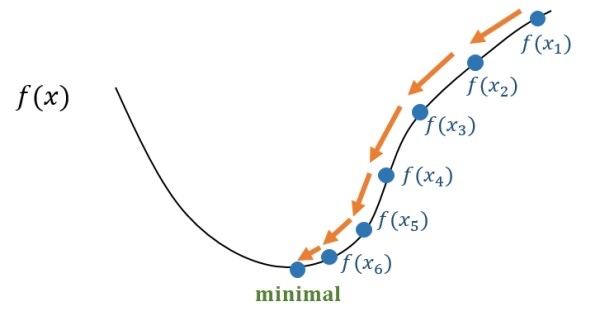\begin{aligned} x_2 &= x_1 + \eta \cdot s_1 \ x_3 &= x_2 + \eta \cdot s_2 \ \vdots \ x_n &= x_{n-1} + \eta \cdot s_{n-1} \end{aligned}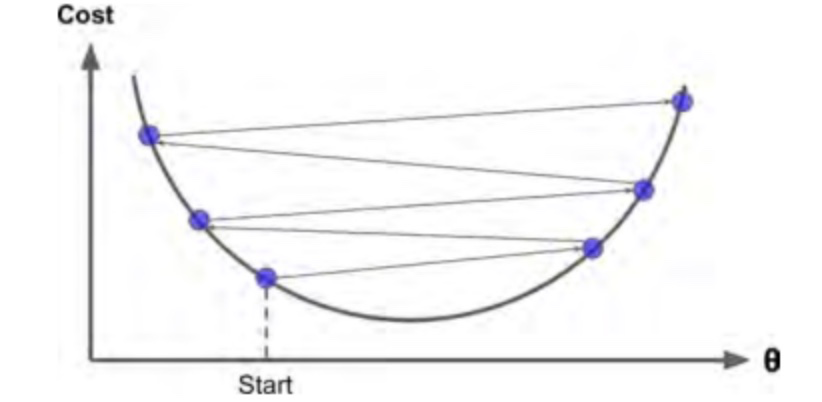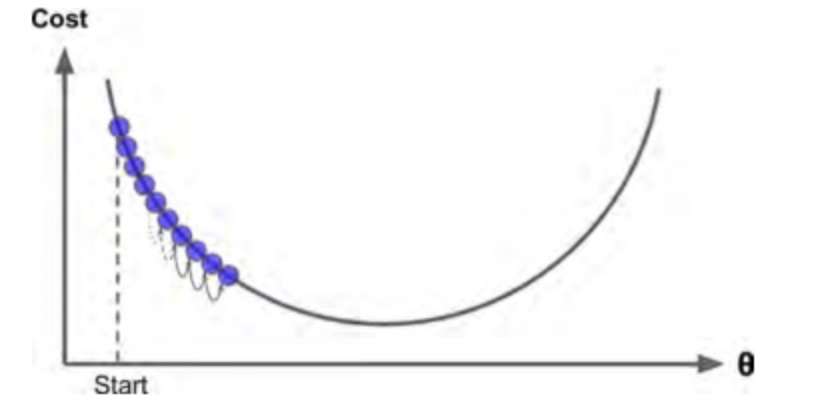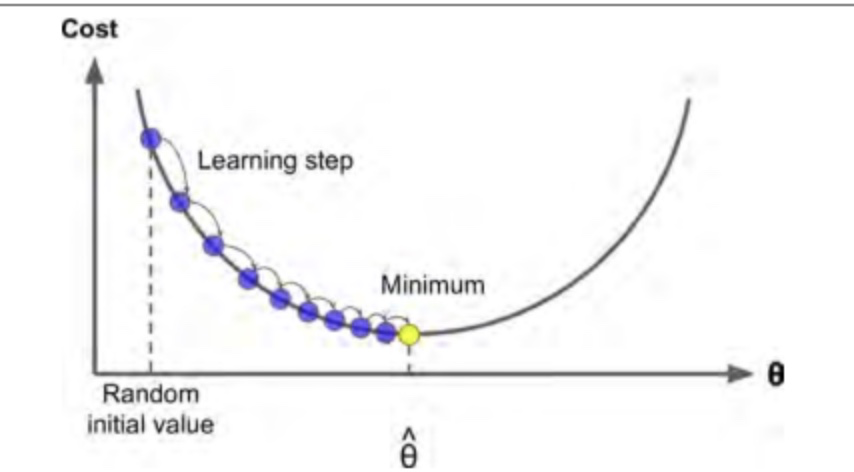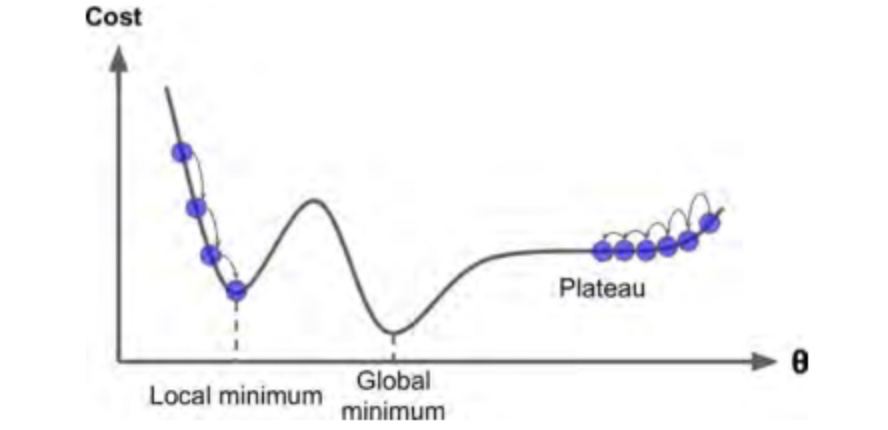<br>

## 代码实战

<br>

Talk is cheap, show me the code.

import numpy as np
X = 2 * np.random.rand(100, 1)
y = 4 + 3 * X + np.random.randn(100, 1)


$\frac {\partial}{\partial \theta_j}MSE(\theta)=\frac {1}{m}\sum_{i=1}^m(\theta^T \cdot x_i-y_i)x_j$

$\nabla_\theta MSE(\theta)=\begin{pmatrix} \frac {\partial}{\partial \theta_0}MSE(\theta) \ \frac {\partial}{\partial \theta_1}MSE(\theta) \ \vdots\ \frac {\partial}{\partial \theta_n}MSE(\theta) \ \end{pmatrix}=\frac {1}{m}X^T\cdot(X \cdot\theta-y)$

$\theta^{next step}=\theta-\eta\nabla_\theta MSE(\theta)$

eta = 0.1 # 学习率
n_iterations = 1000 # 迭代次数
m = 100

theta = np.random.randn(2,1) # 随机初始值
X = np.c_[np.ones(100).T, X]
for iteration in range(n_iterations):
gradients = 1/m * X.T.dot(X.dot(theta) - y) # 根据梯度公式迭代
theta = theta - eta * gradients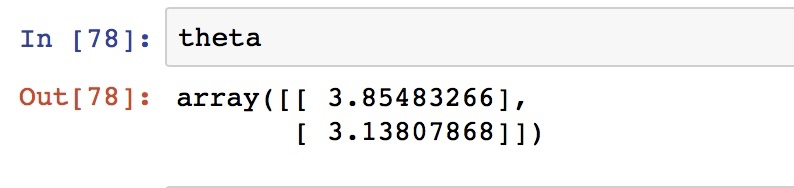### 作者的其它热门文章

0
0 收藏

0 评论
0 收藏
0